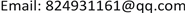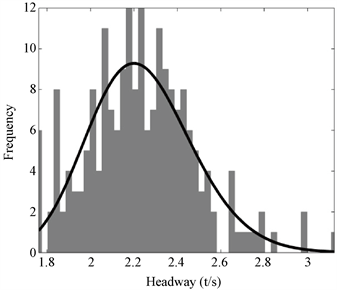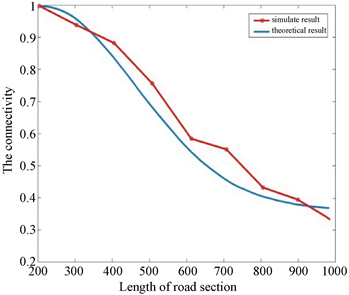﻿ 基于移动属性的车队与路侧单元连通度分析 Analysis of the Connectivity for Platoon-Based Vehicle-to-RSU by Traffic Property

Open Journal of Transportation Technologies
Vol. 09  No. 03 ( 2020 ), Article ID: 35712 , 7 pages
10.12677/OJTT.2020.93026

Analysis of the Connectivity for Platoon-Based Vehicle-to-RSU by Traffic Property

Mingsha Li, Zhiping Zhou, Jiasheng Kang

School of Architecture and Transportation, Guilin University of Electronic Technology, Guilin GuangxiReceived: May 4th, 2020; accepted: May 15th, 2020; published: May 22nd, 2020ABSTRACT

In view of the connection between vehicles and the roadside units in the Vehicular Ad Hoc Network, taking the platoon as the research unit, by studying the traffic property of the vehicles arriving on the road, the connectivity probability analysis had transformed into the probability calculation of the arrived platoon length. This paper proposed that when vehicles are driving in a platoon, the headways of vehicles were subject to a lognormal distribution, in the case of large traffic flow, and then deduced the probability model of the platoon length by convolution formula, and the results verified the correctness of the proposed probability model.1. 引言

2. 连通模型Figure 1. Model of the connectivity for vehicle-to-RSU

3. 车队长度概率模型

3.1. 车头时距分布特性

$P\left(n\right)=\frac{{\left(\lambda t\right)}^{n}{\text{e}}^{-\lambda t}}{n!}$ (1)

$f\left(h (2)

$f\left(t\right)=\left\{\begin{array}{l}\lambda {\text{e}}^{-\lambda \left(t-\Delta \right)},\text{\hspace{0.17em}}\text{\hspace{0.17em}}\text{ }\text{\hspace{0.17em}}t\ge \Delta \\ 0,\text{\hspace{0.17em}}\text{\hspace{0.17em}}\text{\hspace{0.17em}}\text{\hspace{0.17em}}\text{\hspace{0.17em}}\text{\hspace{0.17em}}\text{\hspace{0.17em}}\text{\hspace{0.17em}}\text{\hspace{0.17em}}\text{\hspace{0.17em}}\text{\hspace{0.17em}}\text{\hspace{0.17em}}\text{\hspace{0.17em}}t<\Delta \end{array}$ (3)

$f\left(t\right)=\frac{1}{\sigma \sqrt{2\pi }t}{\text{e}}^{-{\left(\frac{\mathrm{ln}t-\mu }{2\sigma }\right)}^{2}}$ (4)

${f}_{d}\left(d\right)=f\left(t\cdot v\right)=\frac{1}{\sigma \sqrt{2\pi }t\cdot v}{\text{e}}^{-{\left[\frac{\mathrm{ln}t\cdot v-\mu }{2\sigma }\right]}^{2}}$ (5)

3.2. 车队长度概率

${f}_{k}\left(x\right)=A\mathrm{sin}\left(\omega \cdot t\cdot v\right)$ (6)

$f\left({d}_{2}\right)={f}_{d}\ast {f}_{k}={\int }_{{d}_{\mathrm{min}}}^{{d}_{\mathrm{max}}}{f}_{d}\left({d}_{2}\right){f}_{k}\left({d}_{2}-x\right)\text{d}{d}_{2}$ (7)

$\left\{\begin{array}{l}{d}_{\mathrm{min}}=\stackrel{¯}{l}+\alpha \\ {d}_{\mathrm{max}}=\stackrel{¯}{l}+\beta \end{array}$ (8)

$f\left({d}_{n}\right)={\int }_{{d}_{\mathrm{min}}}^{{d}_{\mathrm{max}}}\cdots {\int }_{{d}_{\mathrm{min}}}^{{d}_{\mathrm{max}}}{f}_{d}\left({d}_{2}\right){f}_{d}\left({d}_{3}\right)\cdots {f}_{d}\left({d}_{n}\right){f}_{k}\left(x-{d}_{2}-{d}_{3}-\cdots -{d}_{n}\right)\text{d}{d}_{2}\text{d}{d}_{3}\cdots \text{d}{d}_{n}$ (9)

3.3. 连接概率

${P}_{n}=1-\left[{\int }_{0}^{L-{l}_{n}}{f}_{e}\left(y\right){\int }_{0}^{{l}_{n}}{f}_{{d}_{n}}\left(x\right){f}_{k}\left(L-x\right)\text{d}x\text{d}y+{\int }_{L-{l}_{n}}^{L}{f}_{e}\left(y\right){\int }_{0}^{{l}_{n}}{f}_{{d}_{n}}\left(x\right){f}_{k}\left(L-x\right)\text{d}x\text{d}y\right]$ (10)

$\Delta {P}_{n}\left({l}_{n},L\right)={P}_{n}\left({l}_{n}+\frac{\Delta }{2},L\right)-{P}_{n}\left({l}_{n}-\frac{\Delta }{2},L\right)$ (11)

4. 实例分析

4.1. 车辆移动属性分析Figure 2. Distribution fitting of headway

4.2. 车队与路侧单元连通度分析Figure 3. Comparison of practical and theoretical results of connectivity

5. 结语

(1) 一般运行状态下的车辆到达间隔分布并不符合车队中车辆间隔的分布，分析得出车辆在密集行驶状态下的车头时距符合对数正态分布的结论。

(2) 本文结合提出的车头时距分布，利用卷积公式建立了车队长度概率模型，采用平衡更新理论描述车队中车辆的间隔，在提出的模型中考虑了车辆跟驰行为特性对车辆间隔的扰动，将车队与路侧单元的连通概率计算转化为车队长度概率计算，并通过实例分析验证了模型计算结果与实际基本一致，该研究结果可对路侧单元的部署间隔提供一定的参考依据。

Analysis of the Connectivity for Platoon-Based Vehicle-to-RSU by Traffic Property[J]. 交通技术, 2020, 09(03): 216-222. https://doi.org/10.12677/OJTT.2020.93026

1. 1. 张莉华, 张得生. 基于连接概率的VANETs路由协议研究[J]. 现代电子技术, 2016, 39(7): 19-23.

2. 2. 臧冠男, 沈继伟, 林洋. 车载网中面向高速场景的路由算法[J]. 现代电子技术, 2018, 41(4): 164-168.

3. 3. 王振宇. 车载自组织网络路侧单元部署方法研究[D]: [硕士学位论文]. 南京: 东南大学, 2017.

4. 4. Wang, Y., Jun, Z. and Nathalie, M. (2015) Delivery Delay Analysis for Roadside Unit Deployment in Intermittently Connected VANETs. Global Communications Conference, Austin, 8-12 December 2014, 155-161. https://doi.org/10.1109/GLOCOM.2014.7036800

5. 5. 朱利旻. 车载自组织网络中RSU部署和控制方案的研究[D]: [硕士学位论文]. 南京: 东南大学, 2015.

6. 6. Shao, C., Leng, S. and Zhang, Y. (2015) Performance Analysis of Connectivity Probability and Connectivity-Aware MAC Protocol Design for Platoon-Based VANETs. IEEE Transactions on Vehicular Technology, 64, 5596-5609. https://doi.org/10.1109/TVT.2015.2479942

7. 7. 段力, 过秀成, 姚崇富. 车头时距分布函数的验证分析与选择[J]. 公路交通科技, 2014, 31(5): 147-152.

8. 8. 李全艳, 詹晓松. Hoefs标定下GM跟驰模型仿真分析[J]. 大连交通大学学报, 2014, 35(5): 95-99.

9. 9. TAPAS (2011) “TAPAS Cologne” Scenario. http://kolntrace.project.citi-lab.fr/